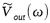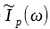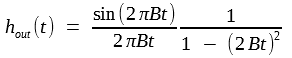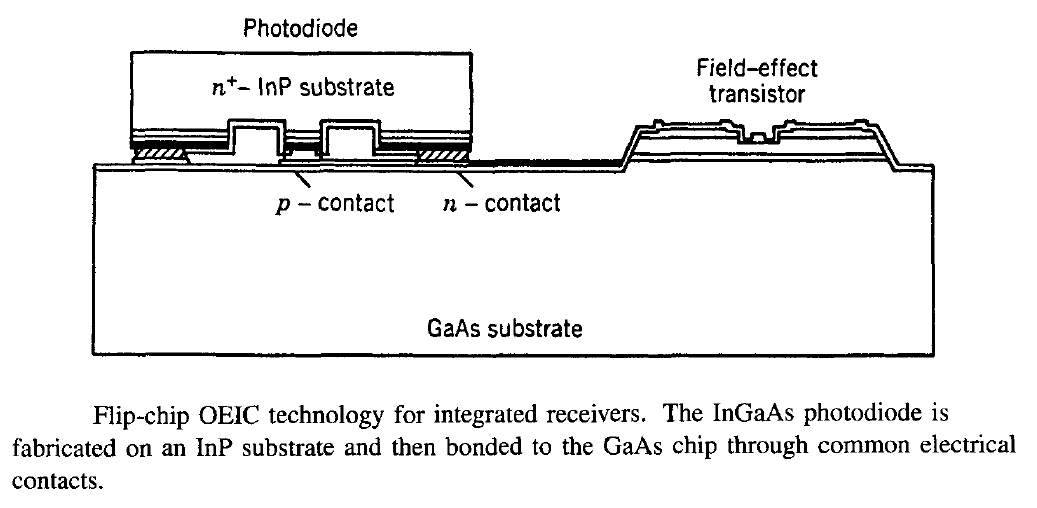The design of an optical receiver depends on the modulation format used by the transmitter. Since most lightwave systems employ the binary intensity modulation, we focus on digital optical receivers. The figure below shows a block diagram of such a receiver. Its components can be arranged into three groups - the front end, the linear channel, and the decision circuit.#### 1. Front End

The front end of a receiver consists of a photodiode followed by a preamplifier. The optical signal is coupled onto the photodiode by using a coupling scheme similar to that used for optical transmitters; butt coupling is often used in practice. The photodiode converts the optical bit stream into an electrical time-varying signal. The role of the preamplifier is to  amplify the electrical signal for further processing.

The design of the front end requires a trade-off between speed and sensitivity. Since the input voltage to the preamplifier can be increased by using a large load resistor RL, a high-impedance front end is often used (see figure (a) below).Furthermore, a large RL reduces the thermal noise and improves the receiver sensitivity. The main drawback of high-impedance front end is its low bandwidth given by Δf = (2πRLCT)-1, where RS << RL is assumed and CT = Cp + CA is the total capacitance, which includes the contributions from the photodiode (Cp) and the transistor used for amplification (CA). The receiver bandwidth is limited by its slowest component. A high-impedance front end cannot be used if Δf is considerably less than the bit rate. An equalizer is sometimes used to increase the bandwidth. The equalizer acts as a filter that attenuates low-frequency components of the signal more than the high-frequency components, thereby effectively increasing the front-end bandwidth. If the receiver sensitivity is not of concern, one can simply decrease RL to increase the bandwidth, resulting in a low-impedance front end.

Transimpedance front ends provide a configuration that has high sensitivity together with a large bandwidth.  Its dynamic range is also improved compared with high-impedance front ends. As seen in figure (b) above, the load resistor is connected as a feedback resistor around an inverting amplifier. Even though RL is large, the negative feedback reduces the effective input impedance by a factor G, where G is the amplifier gain. The bandwidth sis thus enhanced by a factor of G compared with high-impedance front ends. Transimpedance front ends are often used in optical receivers because of their improved characteristics. A major design issue is related to the stability of the feedback loop.

#### 2. Linear Channel

The linear channel in optical receivers consists of a high-gain amplifier (the main amplifier) and a low-pass filter. An equalizer is sometimes included just before the amplifier to correct for the limited bandwidth of the front end. The amplifier gain is controlled automatically to limit the average output voltage to a fixed level irrespective of the incident average optical power at the receiver. The low-pass filter shapes the voltage pulse. Its purpose is to reduce the noise without introducing much intersymbol interference (ISI). The receiver noise is proportional to the receiver bandwidth and can be reduced by using a low-pass filter whose bandwidth Δf is smaller than the bit rate. Since other components of the receiver are designed to have a bandwidth larger than the filter bandwidth, the receiver bandwidth is determined by the low-pass filter used in the linear channel. For Δf < B, the electrical pulse spreads beyond the allocated bit slot. Such a spreading can interfere with the detection of neighboring bits, a phenomenon referred to as ISI.

It is possible to design a low-pass filter in such a way that ISI is minimized. Since the combination of preamplifier, main amplifier, and the filter acts as a linear system (hence the name linear channel), the output voltage can be written aswhere Ip(t) is the photocurrent generated in response to the incident optical power (Ip = RdPin). In the frequency domain,where ZT is the total impedance at the frequency ω and a tilde represents the Fourier transform. Here, ZT(ω) is determined by the transfer functions associated with various receiver components and can be written as

ZT(ω) = Gp(ω)GA(ω)HF(ω)/Yin(ω)

where Yin(ω) is the input admittance and and Gp(ω), GA(ω)HF(ω) are transfer functions of the preamplifier, the main amplifier, and the filter. It is useful to isolate the frequency dependence ofandthrough normalized spectral functions Hout(ω) and Hp(ω), where are related to the Fourier transform of the output and input pulse shapes, respectively, and write the equation above as

Hout(ω) = HT(ω)Hp(ω)

where HT(ω) is the total transfer function of the linear channel and is related to the total impedance as HT(ω) = ZT(ω)/ZT(0). If the amplifiers have a much larger bandwidth than the low-pass filter, HT(ω) can be approximated by HF(ω).

The ISI is minimized when Hout(ω) corresponds to the transfer function of a raised-cosine filter and is given bywhere f = ω/2π and B is the bit rate. The impulse response, obtained by taking the Fourier transform of Hout(f), is given byThe functional form of hout(t) corresponds to the shape of the voltage pulse Vout(t) received by the decision circuit. At the decision instant t = 0, hout(t) = 1, and the signal is maximum. At the same time, hout(t) = 0 for t = m/B, where m is an integer. Since t = m/B corresponds to the decision instant of the neighboring bits, the voltage pulse of the equation above does not interfere with the neighboring bits.

The linear-channel transfer function HT(ω) that will result in output pulse shapes of the form of the equation above is obtained and is given by

HT(f) = Hout(f)/Hp(f)

For an ideal bit stream in the nonreturn-to-zero (NRZ) format (rectangular input pulses of during TB = 1/B), Hp(f) = Bsin(πf/B)/πf, and HT(f) becomes

HT(f) = (πf/2B)cot(πf/2B)

This equation determines the frequency response of the linear channel that would produce output pulse shape given by hout(t) above under ideal conditions. In practice, the input pulse shape is far from being rectangular. The output pulse shape also deviates from hout(t) from above equation, and some ISI invariably occurs.

#### 3. Decision Circuit

The data-recovery section of optical receivers consists of a decision circuit and a clock-recovery circuit. The purpose of the latter is to isolate a spectral component at f = B from the received signal. This component provides information about the bit slot (TB = 1/B) to the decision circuit and helps to synchronize the decision process. In the case of RZ (return-to-zero) format, a spectral component at f = B is present in the received signal; a narrow-bandpass filter such as a surface-acoustic-wave filter can isolate this component easily. Clock recovery is more difficult in the case of NRZ format because the signal received lacks a spectral component at f = B. A commonly used technique generates such a component by squaring and rectifying the spectral component at f = B/2 that can be obtained by passing the received signal through a high-pass filter.

The decision circuit compares the output from the linear channel to a threshold level, at sampling times determined by the clock-recovery circuit, and deices whether the signal corresponds to bit 1 or bit 0. The best sampling time corresponds to the situation in which the signal level difference between 1 and 0 bits is maximum. It can be determined from the eye diagram formed by superposing 2-3-bit-long electrical sequences in the bit stream on top of each other. The figure below shows an ideal eye diagram together with a degraded one in which the noise and the timing jitter lead to a partial closing of the eye. The best sampling time corresponds to maximum opening of the eye.Because of noise inherent in any receiver, there is always a finite probability that a bit would be identified incorrectly by the decision circuit. Digital receivers are designed to operate in such a way that the error probability is quite small (typically < 10-9). Issues related to receiver noise and decision errors are discussed in another tutorial. The eye diagram provides a visual way of monitoring the receiver performance: Closing of the eye is an indication that the receiver is not performing properly.

All receiver components, with the exception of the photodiode, are standard electrical components and can be easily integrated on the same chip by using the integrated-circuit (IC) technology developed for microelectronic devices. Integration is particularly necessary for receivers operating at high bit rates. By 1988, both Si and GaAs IC technologies have been used to  make integrated receivers up to a bandwidth of more than 2 GHz. Since then, the bandwidth has been extended to 10 GHz.

Considerable effort has been directed at developing monolithic optical receivers that integrate all components, including the photodetector, on the same chip by using the optoelectronic integrated-circuit (OEIC) technology. Such a complete integration is relatively easy for GaAs receivers, and the technology behind GaAs-based OEICs is quite advanced. The use of MSM photodiodes has proved especially useful as they are structurally compatible with the well-developed field-effect-transistor (FET) technology. This technique was used as early as 1986 to demonstrate a four-channel OEIC receiver chip.

For lightwave systems operating in the wavelength range 1.3-1.6 μm, InP-based OEIC receivers are needed. Since the IC technology for GaAs is much more mature than for InP, a hybrid approach is sometimes used for InGaAs receivers. In this approach, called flip-chip OEIC technology, the electronic components are integrated on a GaAs chip, whereas the photodiode is made on top of an InP chip. The two chips are then connected by flipping the InP chip on the GaAs chip, as shown in the figure below.The advantage of the flip-chip technique is that the photodiode and the electrical components of the receiver can be independently optimized while keeping the parasitics (e.g., effective input capacitance) to a bare minimum.

The InP-based IC technology has advanced considerably during the 1990s, making it possible to develop InGaAs OEIC receivers. Several kinds of transistors have been used for this purpose. In one approach, a p-i-n photodiode is integrated with the FETs or high-electron-mobility transistors (HEMTs) side by side on an InP substrate. By 1993, HEMT-based receivers were capable of operating at 10 Gb/s with high sensitivity. The bandwidth of such receivers has been increased to >40 GHz, making it possible to use them at bit rates above 40 Gb/s. A waveguide p-i-n photodiode has also been integrated with HEMTs to develop a two-channel OEIC receiver.

In another approach, the heterojunction-bipolar transistor (HBT) technology is used to fabricate the p-i-n photodiode within the HBT structure itself through a common-collector configuration. Such transistors are often called heterojunction phototransistors. OEIC receivers operating at 5 Gb/s (bandwidth Δf = 3 GHz) were made by 1993. By 1995, OEIC receivers making use of the HBT technology exhibited a bandwidth of up to 16 GHz, together with a high gain. Such receivers can be used at bit rates of more than 20 Gb/s. Indeed, a high-sensitivity OEIC receiver module was used in 1995 at a bit rate of 20 Gb/s in a 1.55-μm lightwave system. Even a decision circuit can be integrated within the OEIC receiver by using the HBT technology.

A third approach to InP-based OEIC receivers integrates a MSM or a waveguide photodetector with an HEMT amplifier. By 1995, a bandwidth of 15 GHz was realized for such an OEIC by using modulation-doped FETs. By 2000, such receivers exhibited bandwidths of more than 45 GHz with the use of waveguide photodiode. The following figure shows the frequency response together with the epitaxial-layer structure of such an OEIC receiver. This receiver had a bandwidth of 46.5 GHz and exhibited a responsivity of 0.62 A/W in the 1.55-μm wavelength region. It had a clear eye opening at bit rates of up to 50 Gb/s.Similar to the case of optical transmitters, packaging of optical receivers is also an important issue. The fiber-detector coupling issue is quite critical since only a small amount of optical power is typically available at the photodetector. The optical-feedback issue is also important since unintentional reflections fed back into the transmission fiber can affect system performance and should be minimized. In practice, the fiber tip is cut at an angle to reduce the optical feedback. Several different techniques have been used to produce packaged receivers capable of operating at bit rates as high as 10 Gb/s. In one approach, an InGaAs APD was bonded to the Si-based IC by using the flip-chip technique. Efficient fiber-APD coupling was realized by using a slant-ended fiber and a microlens monolithically fabricated on the photodiode. The fiber ferrule was directly laser welded to the package wall with a double-ring structure for mechanical stability. The resulting receiver module withstood shock and vibration tests and had a bandwidth of 10 GHz.

Another hybrid approach makes use of a planar-lightwave-circuit platform containing silica waveguides on a silicon substrate. In one experiment, an InP-based OEIC receiver with two channels was flip-chip bonded to the platform. The resulting module could detect two 10-Gb/s channels with negligible crosstalk. GaAs ICs have also been used to fabricate a compact receiver module capable of operating at a bit rate of 10 Gb/s. By 2000, fully packaged 40-Gb/s receivers were available commercially. For local-loop applications, a low-cost package is needed. Such receivers operate at lower bit rates but they should be able to perform well over a wide temperature range extending from -40 to 85°C.